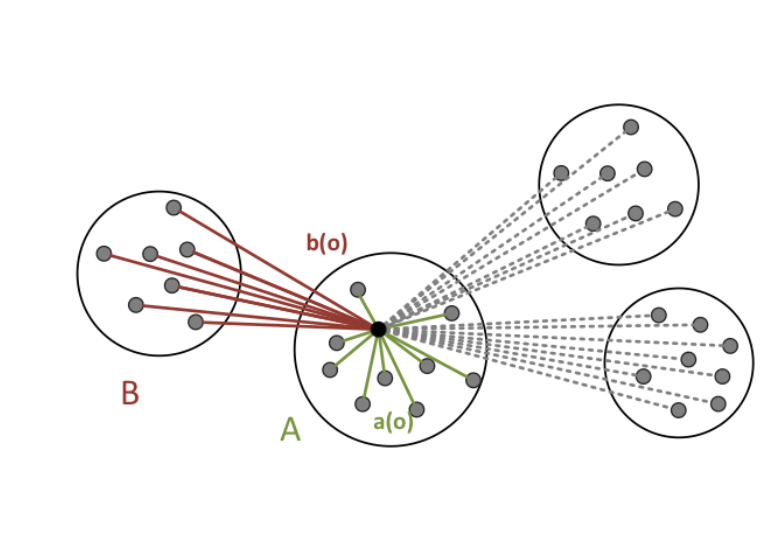# Clustering – Partitioning Methods

4_1_Partitioning_Methods

## 定义

### Partitioning method

• 给每个簇挑选$k$$k$个代表，可以随机挑选或者用其他算法。

• 递归地改善刚刚挑选到的簇：

• 在当前的聚类(clustering)下，把每个对象分配到最合适的簇(cluster)中
• 基于刚才的重新聚类，重新计算新的簇的代表。
• 重复到不再改变

Given a set $D$$D$, a partitioning $\mathcal{C}=\left\{{C}_{1},...,{C}_{k}\right\}$$\mathcal{C}=\{C_1,...,C_k\}$ of $D$$D$ fulfills:

• ${C}_{i}\subseteq D$$C_i\subseteq D$ for all $1\le i\le k$$1\leq i \leq k$
• ${C}_{i}\cap {C}_{j}=\mathrm{\varnothing }⇔i\ne j$$C_i\cap C_j = \empty \Leftrightarrow i\neq j$
• $\bigcup {C}_{i}=D$$\bigcup C_i=D$

i.e. each element of $D$$D$ is in exactly one set ${C}_{i}$$C_i$.

## K-mean

K-mean 算法的目的是：

Find a clustering such that the within-cluster variation of each cluster is small and use the centroid of a cluster as a representative.

### 符号规定

• 对象(objects) $\mathbf{\text{p}}=\left({p}_{1},...,{p}_{d}\right)$$\textbf{p}=(p_1,...,p_d)$ 是在一个$d$$d$维向量空间的点。

• 平方距离和（用来衡量一个簇的紧凑程度(compactness))(sum of squared distances):

$\begin{array}{}\text{(1)}& SSE\left({C}_{j}\right)=\sum _{\mathbf{\text{p}}\in {C}_{j}}||\mathbf{\text{p}}-{\mu }_{{C}_{j}}|{|}_{2}^{2}\end{array}$

其中${C}_{j}$$C_j$表示一个簇,${\mu }_{{C}_{j}}$$\mu_{C_j}$表示簇${C}_{j}$$C_j$的平均值。

• 对于整个聚类$\mathcal{C}$$\mathcal{C}$的紧凑程度:

$\begin{array}{}\text{(2)}& SSE\left(\mathcal{C}\right)=\sum _{{C}_{j}\in \mathcal{C}}SSE\left({C}_{j}\right)=\sum _{\mathbf{\text{p}}\in D}||\mathbf{\text{p}}-{\mu }_{{C}_{j}}|{|}_{2}^{2}\end{array}$

也就是整个数据集$D$$D$中所有点的$SSE$$SSE$之和。

有的书上也用簇内变差$E$$E$来表示。假设聚类clustering $\mathcal{C}$$\mathcal{C}$中一共有k个簇，那么

$\begin{array}{}\text{(3)}& E=\sum _{i=1}^{k}\sum _{\mathbf{\text{p}}\in {C}_{i}}dist\left(\mathbf{\text{p}},{\mathbf{c}}_{\mathbf{i}}{\right)}^{2}\end{array}$

其中$dist\left(p,{c}_{i}\right)$$dist(p,c_i)$函数表示点$\mathbf{p}$$\mathbf{p}$${\mathbf{c}}_{\mathbf{i}}$$\mathbf{c_i}$之间的欧式距离,这两个点都是多维的。这里(2)式和(3)式的意思完全一致

• 最佳划分: $\mathrm{arg}\underset{\mathcal{C}}{min}SSE\left(\mathcal{C}\right)$$\arg\min_{\mathcal{C}} SSE(\mathcal{C})$ 使得整体SSE最小的聚类。

### 算法

k-mean算法则是利用贪心算法，将复杂度尽可能的降低。算法首先在数据集中随机选择$k$$k$个对象，每个对象都代表一个簇的初始均值或者中心。对于剩下的对象，根据其与各个簇中心的欧式距离，将它分配到最相似的簇。接下来，k-mean算法迭代地改善$SSE$$SSE$：对于每个簇，算法使用上次迭代分配到的该簇的对象，计算新的均值。然后用更新后的均值作为新的形心，重新分配所有对象。一直迭代到本轮形成的簇和上一轮一样。

1. 给定$k$$k$作为簇的数目
2. 给定包含$n$$n$个对象的数据集$D$$D$

1. 挑选k个随机代表对象${p}_{1},{p}_{2},...{p}_{k}$$p_1,p_2,...p_k$

1. $D$$D$中每个对象分配到离之最近的代表对象${p}_{i}$$p_i$中,$i\in 1,...,k$$i\in1,...,k$
2. 为每个簇重新计算形心，并把每个形心作为新的代表对象

1. 代表对象不再改变

### 分析

k-mean算法复杂度是$O\left(nkt\right)$$O(nkt)$，n是对象总数，k是簇数，t是迭代次数。通常情况下有$k<$k<.所以对于大数据集这种算法非常有效。

1. 算法复杂度通常情况下比较低
2. 易于部署。

1. 必须给出簇的均值的定义。
2. 要求用户事前给出$k$$k$
3. 对噪声和离群点敏感。因为少量的极值会对均值产生很大影响。
4. 簇要求形状得是凸的
5. 性能或者说是时间复杂度与初始化的关系很大。

## k-Median

k-mean算法对离群点比较敏感。改进的一种想法是不让对象和均值比较，而是让对象和对象比较。

$SSE\left(\mathcal{C}\right)$$SSE(\mathcal{C})$类似，这里我们可以定义一个绝对误差标准(absolute-error criterion):

$\begin{array}{}\text{(4)}& AEC\left(\mathcal{C}\right)=\sum _{{C}_{j}\in \mathcal{C}}AEC\left({C}_{j}\right)=\sum _{\mathbf{\text{p}}\in D}||\mathbf{\text{p}}-{\mathbf{o}}_{\mathbf{i}}|{|}_{2}^{2}\end{array}$

$\begin{array}{}\text{(5)}& E=\sum _{i=1}^{k}\sum _{\mathbf{p}\in {C}_{j}}dist\left(\mathbf{p},{\mathbf{o}}_{\mathbf{i}}\right)\end{array}$

k-median算法同样可以计算无法取均值的ordering数据。

k-median算法与k-mean类似，只是公式不同，在此不做赘述，有兴趣可以阅读这篇文章，介绍了k-mean,k-median，以及衍生出的PAM

## Silhouette-Coefficient

Silhouette-Coefficient可以评估一个聚类的质量。挑选它的原因也很简单，这个系数不会因为k的增加而单调。

Silhouette-Coefficient的想法是，聚类的质量好坏，对应于这个聚类是否将对象“恰当”得映射到了聚类里。所谓恰当，正如我们前面提到的，就是想让同一个簇内的对象尽可能相似，不同的簇的对象尽可能相异。那么我们就可以规定$a\left(o\right)$$a(o)$来表示代表对象$o$$o$与簇内其他对象的相似性，用$b\left(o\right)$$b(o)$表示相异性。

$\begin{array}{}\text{(6)}& a\left(o\right)=\frac{1}{|C\left(o\right)|}\sum _{\mathbf{p}\in C\left(o\right)}dist\left(\mathbf{o},\mathbf{p}\right)\end{array}$

$|C\left(o\right)|$$|C(o)|$表示代表对象$o$$o$所对应簇$C\left(o\right)$$C(o)$的对象的数量。

$b\left(o\right)$$b(o)$可以用代表对象到其他簇的对象的最小距离的平均值来表示

$\begin{array}{}\text{(7)}& b\left(o\right)=\underset{{C}_{i}\ne C\left(o\right)}{min}\left\{\frac{1}{|{C}_{i}|}\sum _{p\in {C}_{i}}d\left(o,p\right)\right\}\end{array}$Silhouette Coefficient定义如下:

$\begin{array}{}\text{(9)}& s\left({C}_{i}\right)=\frac{1}{|{C}_{i}|}\sum _{o\in {C}_{i}}s\left(o\right)\end{array}$

$\begin{array}{}\text{(10)}& s\left(\mathcal{C}\right)=\frac{1}{|D|}\sum _{o\in D}s\left(o\right)\end{array}$

$D$$D$表示整个数据集

## Reference

 S.P. Lloyd: Least squares quantization in PCM. In IEEE Information Theory, 1982

## 2条评论

1.小熊说道：

又是一个我插不上嘴的博客，太专业了

1.Mhrooz说道：

谢谢回复！

这里写的也只是一些皮毛罢了。

你的导航站也很棒啊，很开心能遇到这么多2022依然在坚持用博客的人:)